# Multiplying Mixed Numbers

Method One:

One method for multiplying mixed numbers is to change them to improper fractions.

Here are some examples.

Problem #1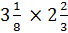Step 1 : Change each number to an improper fraction.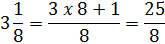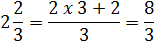Step 2: Multiply the numerators. Then multiply the denominators.In this example, we have common factors on the top and bottom that can be canceled out.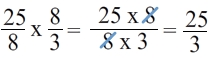So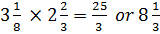Let's try those steps again.

Problem #2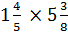Step 1: Change the mixed numbers to improper fractions.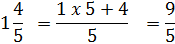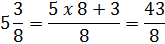Step 2: Multiply.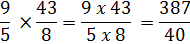You can leave the answer like this or you can rewrite it as a mixed number.

387 ÷ 40 = 9Method 2:

Using an area model.

If you like drawing diagram to help you determine solutions, then you might like this method better.

Problem #3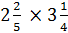Start by drawing a model that has a spot for the whole number part and a spot for the fraction part of each mixed number.Next, we will multiply each row with each column.Last, we add up all the products inside the table.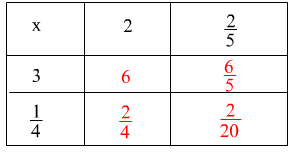Although you did not have to change to improper fractions, you do have to add fractions with unlike denominators.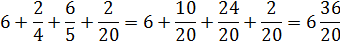Now we have an improper fraction in our mixed number.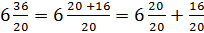=7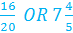This method still requires quite a bit of work and could be difficult if the common denominators get too large. However, it will work and if you find that it works for you, the go with it!

Math
Fractions
Fraction Multiplication Worksheets
Mixed number to improper fraction worksheets
Improper Fractions and Mixed number worksheets
Convert Decimals to Fractions Quiz
Add and Subtract Like Fractions Quiz
Add and Subtract unlike fractions Quiz
Multiply Fractions Quiz
Dividing Fractions Quiz
Factors